• +91 9971497814
• info@interviewmaterial.com

# RD Chapter 10- Congruent Triangles Ex-10.1 Interview Questions Answers

### Related Subjects

Question 1 :
Write the complement of each of the following angles:
(i) 20°
(ii) 35°
(iii) 90°
(iv) 77°
(v) 30°

We know that two angles are complement to each other if their sum is 90°. Therefore,
(i) Complement of 20° is (90° – 20°) = 70°
(ii) Complement of 35° is (90° – 35°) = 55°
(iii) Complement of 90° is (90° – 90°) = 0°
(iv) Complement of 77° is (90° – 77°) = 13°
(v) Complement of 30° is (90° – 30°) = 60°

Question 2 :
Write the supplement of each of the following angles:
(i) 54°
(ii) 132°
(iii) 138°

We know that two angles are supplement to each other if their sum if 180°. Therefore,
(i) Supplement of 54° is (180° – 54°) = 126°
(ii) Supplement of 132° is (180° – 132°) = 48°
(iii) Supplement of 138° is (180° – 138°) = 42°

Question 3 : If an angle is 28° less than its complement, find its measure.

Let required angle = x, then
Its complement = x + 28°
∴  x + x + 28° = 90° ⇒  2x = 90° – 28° = 62°
∴ x =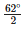= 31°
∴ Required angle = 31°

Question 4 : If an angle is 30° more than one half of its complement, find the measure of the angle.

Let the measure of the required angle = x
∴  Its complement =  90° – x
∴  x =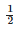(90° – x) + 30°
2x = 90° – x + 60°
⇒ 2x + x = 90° + 60°
⇒  3x = 150°
⇒ x =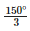= 50°
∴ Required angle = 50°

Question 5 : Two supplementary angles are in the ratio 4 : 5. Find the angles.

Ratio in two supplementary angles = 4 : 5
Let first angle = 4x
Then second angle = 5x
∴  4x + 5x = 180
⇒  9x = 180°
∴ x  =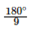= 20°
∴  First angle = 4x = 4 x 20° = 80°
and second angle = 5x
= 5 x 20° = 100°

Question 6 : Two supplementary angles differ by 48°. Find the angles.

Let first angle = x                        ”
Then second angle = x + 48°
∴  x + x + 48° = 180°⇒  2x + 48° = 180°
⇒  2x = 180° – 48° = 132°
x=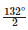=66°
∴  First angle = 66°
and second angle = x + 48° = 66° + 48° = 114°
∴ Angles are 66°, 114°

Question 7 : An angle is equal to 8 times its complement. Determine its measure.

Let the required angle = x
Then its complement angle = 90° – x
∴ x = 8(90° – x)
⇒ x = 720° – 8x ⇒  x + 8x = 720°
⇒ 9x = 720° ⇒ x =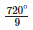= 80°
∴  Required angle = 80°

Question 8 : If the angles (2x – 10)° and (x – 5)° are complementary angles, find x.

First complementary angle = (2x – 10°) and second = (x – 5)°
∴ 2x – 10° + x – 5° = 90°
⇒ 3x – 15° = 90° ⇒  3x = 90° + 15° = 105°
∴ x =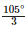= 35°
∴  First angle = 2x – 10° = 2 x 35° – 10°
= 70° – 10° = 60°
and second angle = x – 5 = 35° – 5 = 30°

Question 9 : If an angle differ from its complement by 10°, find the angle.

Let required angle = x°
Then its complement angle = 90° – x°
∴ x – (90° – x) = 10
⇒  x – 90° + x = 10°⇒  2x = 10° + 90° = 100° 100°
⇒ x =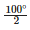= 50°
∴ Required angle = 50°

Question 10 : If the supplement of an angle is two-third of itself Determine the angle and its supplement.

Let required angle = x
Then its supplement angle = 180° – x
∴  (180°-x)=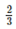x
540° – 3x = 2x ⇒ 2x + 3x = 540°
⇒ 5x = 540°⇒  x =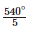= 108°
-. Supplement angle = 180° – 108° = 72°

Todays Deals### RD Chapter 10- Congruent Triangles Ex-10.1 Contributorskrishan

Name:
Email:

# Latest News# 9000 interview questions in different categories